# KR100796735B1 - A method for generating a data structure for a volume ray casting - Google Patents

A method for generating a data structure for a volume ray casting Download PDF

## Info

Publication number
KR100796735B1
KR100796735B1 KR1020070091334A KR20070091334A KR100796735B1 KR 100796735 B1 KR100796735 B1 KR 100796735B1 KR 1020070091334 A KR1020070091334 A KR 1020070091334A KR 20070091334 A KR20070091334 A KR 20070091334A KR 100796735 B1 KR100796735 B1 KR 100796735B1
Authority
KR
South Korea
Prior art keywords
cell
candidate
sub
method
squares
Prior art date
Application number
KR1020070091334A
Other languages
Korean (ko)
Inventor
신병석
임석현
Original Assignee
인하대학교 산학협력단
Priority date (The priority date is an assumption and is not a legal conclusion. Google has not performed a legal analysis and makes no representation as to the accuracy of the date listed.)
Filing date
Publication date
Application filed by 인하대학교 산학협력단 filed Critical 인하대학교 산학협력단
Priority to KR1020070091334A priority Critical patent/KR100796735B1/en
Application granted granted Critical
Publication of KR100796735B1 publication Critical patent/KR100796735B1/en

• 238000000034 methods Methods 0.000 description 11
• 238000004364 calculation methods Methods 0.000 description 5

## Images

•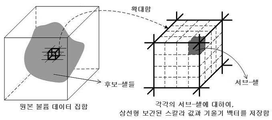•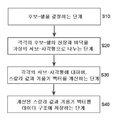•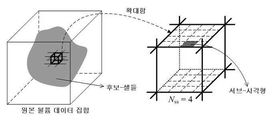•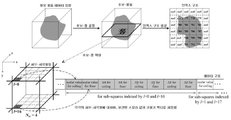••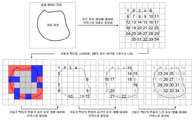•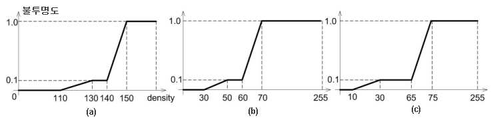•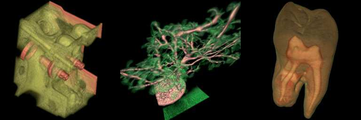## Classifications

• GPHYSICS
• G06COMPUTING; CALCULATING; COUNTING
• G06TIMAGE DATA PROCESSING OR GENERATION, IN GENERAL
• G06T15/003D [Three Dimensional] image rendering
• G06T15/08Volume rendering
• GPHYSICS
• G06COMPUTING; CALCULATING; COUNTING
• G06TIMAGE DATA PROCESSING OR GENERATION, IN GENERAL
• G06T15/003D [Three Dimensional] image rendering
• G06T15/06Ray-tracing
• GPHYSICS
• G06COMPUTING; CALCULATING; COUNTING
• G06TIMAGE DATA PROCESSING OR GENERATION, IN GENERAL
• G06T3/00Geometric image transformation in the plane of the image
• G06T3/40Scaling the whole image or part thereof
• G06T3/4007Interpolation-based scaling, e.g. bilinear interpolation
• GPHYSICS
• G06COMPUTING; CALCULATING; COUNTING
• G06TIMAGE DATA PROCESSING OR GENERATION, IN GENERAL
• G06T2210/00Indexing scheme for image generation or computer graphics
• G06T2210/56Particle system, point based geometry or rendering

## Abstract

A method for creating a data structure for volume ray casting is provided to remarkably shorten rendering time as compared with an existing method by dividing the ceiling and floor of each candidate cell into virtual sub-squares, calculating a scalar value and a gradient vector for each divided sub-square by using bilinear interpolation, and storing them in a data storage. A control part determines candidate cells(S10). The control part divides the ceiling and floor of each of the candidate cells into virtual sub-squares(S20). The control part calculates scalar values and gradient vectors for the divided sub-squares respectively using bilinear interpolation(S30). The control part stores the calculated scalar values and gradient vectors in a data storage(S40).

## Description

A METHOD FOR GENERATING A DATA STRUCTURE FOR A VOLUME RAY CASTING}

The present invention relates to a method of generating a data structure for volume ray casting, and more particularly to the virtual sub- ceiling of each candidate cell. For each sub-square divided by a square, the scalar value and the gradient vector are calculated and stored as a data structure using bilinear interpolation, which is compared with the conventional method. The present invention relates to a method for generating a data structure for a volume ray projection method which can greatly reduce the time and at the same time significantly reduce the time and memory required for preprocessing compared to the conventional method of dividing candidate-cells into sub-cells. .

Volume rendering is a technique of extracting and visualizing meaningful information from volume data. Volume rendering is largely divided into surface rendering and direct volume rendering. Volume ray projection is one of the direct volume rendering techniques and is widely used because it can generate high quality images. Volume ray projection is largely divided into the steps of leaping empty space and calculating color values. The biggest drawback of the volume ray projection method is the slow rendering speed, and many studies to solve this problem have focused on the method of rapidly leaping the empty space that does not contribute to the final image. However, in order to calculate the color value after the empty space, the rendering speed is inevitably deteriorated because the main memory must be referred to several times arbitrarily. This slowing down of rendering speed is more severe when there are many opaque voxels.

Many methods have been proposed to accelerate the CPU-based volume rendering technique. Yagel et al. Proposed a method to shorten the sampling operation by using a template that has previously calculated the position and resampling weight of the sample along the ray propagation path. This method is only available for orthogonal projection. It is disadvantageous in that it is difficult to use in a field requiring perspective projection. Shear-warp volume rendering is a method of accelerating the color-value calculation step by increasing memory reference efficiency, causing cache misses in interactive classification environments, and improving memory reference efficiency. Since the resampling filter uses bilinear interpolation, there is a problem that image quality is degraded. Parker et al. Proposed a method of accelerating rendering speed by improving the memory approach on workstations, which was limited to isosurface rendering and required 128 CPUs for real-time rendering. A volume ray projection system that accelerates cache operations using Intel's MMX TM (MultiMedia eXtension) technology has also been announced, which has a near real-time rendering speed but has a problem that the image size is limited to 256 × 256. . If a higher resolution is desired, the image of 256 × 256 has to be enlarged, but this causes a problem of blurring of the image. Mora et al. Introduced the concept of splatting in volume ray projection to propose an object-based volume ray casting method that projects cells in the same cube shape. There is a problem that cannot be used. A method of solving the shortcomings of the volume ray projection method by using random access to memory references and efficient use of gradient vectors stored in the cache space of the CPU has been introduced. Shows speeds of more than 10 frames per second, but also has the disadvantage that it is only possible for orthogonal projection. In addition, a method of accelerating rendering speed by changing thread-level parallelism (TLP) and access order of volume data has been proposed.

Recently, a data structure for accelerating the color value acquisition speed has been proposed in the volume ray projection method, which divides candidate-cells into virtual N ss three sub-cells during preprocessing, and then three lines for each sub-cell. After storing the scalar values and the gradient vectors computed by trilinear interpolation, they were used in the rendering phase. 1 is a diagram illustrating storing a scalar value and a gradient vector calculated by trilinear interpolation after dividing one candidate-cell into N ss three sub-cells according to the above method. In the example shown in FIG. 1, one candidate-cell is divided into 64 (= 4 3 ) sub-cells. The method has the advantage of reducing the rendering time, but when the number of candidate cells is increased, a large amount of time is required for preprocessing, and the memory consumption is also greatly increased.

The present invention has been proposed to solve the above problems of the conventionally proposed methods, and it is possible to significantly reduce the rendering time as compared to the conventional methods, and at the same time, to save the time and memory required for preprocessing and to serve the candidate-cells. It is an object of the present invention to provide a method for generating a data structure for the volume ray projection method which can be greatly reduced as compared with the conventional method of dividing into cells.

According to a feature of the present invention for achieving the above object, a method for generating a data structure for the volume ray projection method,

(1) determining a candidate cell by checking whether the cell is a transparent cell or not-a cube consisting of eight voxels, which are three-dimensional pixels (cube) structure is called a cell, the case where all eight voxels constituting the cell are transparent is called a transparent cell, the cell that affects the final image is called a candidate-cell-;

(2) for each of the determined candidate-cells, dividing the ceiling and the floor into imaginary sub-squares;

(3) for each of the divided sub-squares, calculating a scalar value and a gradient vector using bilinear interpolation; And

(4) storing the calculated scalar value and the gradient vector as a data structure.

Preferably, in step (4), the index of candidate-cells for referencing the data structure may be stored as an index structure.

Preferably, in step (2), after determining the degree of change of the gradient vector of the candidate-cell, the number of sub-squares divided by the number of sub-squares for the candidate-cell determined that the change of the gradient vector is greater than a predetermined reference value is determined. It is possible to increase the number of sub-squares divided for candidate-cells determined that the change of the gradient vector is smaller than a predetermined reference value.

More preferably, the degree of change of the image of the candidate-cell may be determined using the degree of change of the gradient vector.

According to the data structure generation method for the volume ray projection method of the present invention, the ceiling and the bottom of the candidate-cell are divided into virtual sub-squares, and for each of the divided sub-squares, a scalar value using bilinear interpolation. By calculating and storing the vector and the gradient vector in the data structure, the rendering time can be greatly reduced compared to the conventional method, and at the same time, the time and memory required for the preprocessing are significantly larger than those of the conventional method of dividing the candidate-cell into sub-cells. Can be reduced.

Hereinafter, exemplary embodiments of the present invention will be described in detail with reference to the accompanying drawings.

First, the color value calculation process in the volume ray projection method will be briefly described, and then the data structure generation method according to the present invention will be described in detail.

In the volume ray projection method, the color value calculation process is largely composed of three procedures. (1) First, an accurate scalar value is calculated from eight voxels surrounding a sample point by using a resampling filter to determine an accurate transparency value when a ray is placed at an arbitrary sample point. In the volume ray projection method, since trilinear interpolation is generally used, it has a long processing time and has a problem of arbitrarily referring to the main memory. (2) Second, in order to calculate the gradient vector at the corresponding sample point, the gradient vector is calculated at eight voxels surrounding the sample point. Even the simplest central difference requires about 48 random-accesses to main memory. (3) Thirdly, the eight gradient vectors calculated in the second procedure are obtained by using the three-linear interpolation to obtain the gradient vectors at the corresponding sample points. The desired color is obtained from the gradient vector obtained through the lighting model.

The above process is performed for all sample points in the corresponding ray, and these operations are repeated for every pixel of the image. The most obvious way of accelerating these steps is to precompute the parts that randomly access the main memory in the preprocessing step and refer to the results in the rendering step. The present invention proposes a method of generating a data structure that can store the results calculated in the preprocessing step and refer to it in the rendering step.

2 is a diagram illustrating a method of generating a data structure for a volume ray projection method according to an embodiment of the present invention. As shown in FIG. 2, the method for generating a data structure according to the present invention includes (1) determining a candidate-cell (S10), and (2) virtually sub-curing the ceiling and the floor of each determined candidate-cell. Dividing into squares (S20), (3) calculating the scalar value and the gradient vector for each divided sub-square (S30), and (4) storing the calculated scalar value and the gradient vector in the data structure. It includes the step (S40). In step S10, whether or not any cell is a candidate-cell may be determined by checking whether the cell is transparent-cell. The candidate-cell refers to a cell that affects the final image. When all eight voxels constituting the cell are not transparent transparent cells, the candidate-cell may be determined as a candidate-cell. In operation S20, the number of sub-squares dividing the candidate-cell may be adjusted according to the degree of change of the image of the candidate-cell. That is, the number of sub-squares divided for candidate-cells determined to be large in image change may be increased, and the number of sub-squares divided in candidate-cells determined to be small in image change may be reduced. The degree of change in the image of the candidate-cell may be confirmed by the degree of change in the gradient vector of the candidate-cell. In this regard, it will be described in more detail later with reference to FIG. 6. In step S30, the scalar value and the slope vector may be calculated using bilinear interpolation. In step S40, the index of the candidate-cells may be stored in the index structure to refer to the data structure.

3 illustrates dividing a candidate-cell into sub-squares according to an embodiment of the present invention. A cube consisting of eight voxels is called a cell, and if all eight voxels in one cell are transparent, it is called a transparent cell. A cell that affects the final image is a candidate cell. cell), such as, shown in Figure 3 referred to, in the present invention, each of the candidate-rectangle - the ceiling and the floor of the cell N 2 ss of the virtual rectangle (sub; divided into sub-square). In other words, according to the method of the present invention, a candidate-cell 2N ss 2 of sub-divided into squares. In the embodiment shown in FIG. 3, one candidate-cell is divided into 32 (= 2 × 4 2 ) sub-squares.

After dividing one candidate-cell into sub-squares, the next step calculates the data needed to accelerate the color value calculation step of volume ray projection. For an arbitrary sample point, if the trilinear interpolated scalar value and the gradient vector are stored in advance, the rendering speed can be greatly reduced by reducing the operation of arbitrarily referencing the main memory. As mentioned above, although such a method has been proposed in the past, the method has a problem in that the amount of data required is excessively increased when the number of candidate cells is large. In order to solve this problem, in the present invention, instead of dividing the candidate-cell into several sub-cells, a method of dividing the ceiling and the bottom of the candidate-cell into sub-squares is used. These sub-squares store scalar values and slope vectors computed by bilinear interpolation. When using the method proposed in the present invention, as compared with the conventional method of dividing the candidate-cell into sub-cells, as shown in Equations 1 and 2, the memory can be saved by 2 / N ss . The pretreatment time can be reduced by 1 / N ss .

In the above equations, t lint represents the time taken to perform one linear interpolation.

For each sub-square, scalar values and slope vectors computed by bilinear interpolation are stored in the data structure, which stores the index of candidate cells in order to refer to the stored data structure. Additionally needed. This is called an index structure. In the index structure, null values are stored when the values of the voxels are transparent, and the accumulated indexes are stored. 4 is a diagram illustrating a process of generating a data structure and an index structure according to the method proposed by the present invention. As shown in FIG. 4, an index structure is created that can refer to each candidate-cell, and at the same time divide each candidate-cell into sub-squares, and then linearly for each divided sub-square. By interpolation, scalar values and gradient vectors are calculated and stored in a data structure. Referring to the data structure according to the embodiment of the present invention shown in FIG. 4, the data structure may be generated together for a pair of ceiling and floor, and each of the ceiling and the floor may have a different structure. Scalar values and gradient vectors in each direction for the ceiling and floor may be stored together. In FIG. 4, a ceiling indexed with l = 0 and a floor indexed with l = 16 are stored together, and a ceiling indexed with l = 1 and a floor indexed with l = 17 are stored together.

The memory requirement φ when generating the data structure in this way may be calculated as in Equation 3 below.

Where N cc represents the total number of candidate cells and N 3 represents the size of volume data. The size of the index structure depends on the number of candidate cells, but a 4-byte data structure is usually sufficient.

How the data structure generated in the preprocessing step according to the present invention can be used in the rendering step will be described with reference to FIG. 5 is a diagram illustrating a state in which a data structure generated according to the present invention is used in a rendering step. In the rendering stage, when the viewpoint is fixed, a virtual ray is first emitted from the pixel of the image. After jumping to a clear space, when a particular sample point of any candidate-cell is reached, the sub-squares of the ceiling and the bottom closest to the sample point are selected, as shown in FIG. With respect to scalar values previously calculated by the bilinear interpolation of the corresponding sub-squares, the scalar value calculated by the accurate trilinear interpolation method is obtained by performing one linear interpolation. If it is determined that the value is transparent, it moves to the next sample point, and if it is opaque, it performs three linear interpolation once for each of the x, y, and z components of the pre-calculated bilinear interpolated gradient vector. Find the interpolated slope vector. The gradient value is used to obtain a color value. This operation is repeated until the opacity is less than 1.0, and is performed for all pixels of the image. As can be seen from above, when using the data structure generated according to the present invention, since most operations that arbitrarily refer to the main memory are precomputed, the rendering time can be significantly shortened.

The method proposed in the present invention can reduce the amount of time and memory required for preprocessing compared to the conventional method of dividing candidate-cells into sub-cells. However, when the number of candidate-cells increases, more memory is required. Can face. In order to solve this problem, the present invention uses a variable gradient vector control method together. The variable gradient vector control method increases the number of sub-squares for candidate-cells with a large change in image (i.e. a large change in the gradient vector) in dividing one candidate-cell into a plurality of sub-squares. Otherwise, the number of sub-squares is relatively reduced. According to an embodiment of the present invention, the number of sub-squares is differentially allocated by distinguishing the data into three steps according to a user-defined value.

When the variable slope vector adjustment method is used as described above, the number of sub-squares is changed, so the storage method of the index structure storing them is also different. If the existing method is applied without change, three index structures are required, which increases the memory requirements. In order to solve this problem, in the present invention, after dividing the number of sub-squares into three stages according to the gradient vector, they are sorted using a conventional sorting algorithm and the total candidate cells in each stage. We propose a method of storing the number of. In the rendering step, the data structure is referred to by adding the index stored in the current index structure and the number of candidate cells in each step. In this case, even with one index structure, the variable gradient vector control can be effectively applied. Of course, pre-processing requires one sorting process, but it's a negligible increase in memory requirements because it only scans the entire volume once. FIG. 6 is a diagram illustrating the above description, which shows that the number of sub-squares is divided differently according to the degree of change of the gradient vector.

Experiment result

The proposed method was tested on a system equipped with AMD's Athlon 64 × 2 4200+ CPU and 2GB of main memory. As experimental data, engine block data and bonsai data having a size of 256 3 , and dental data having a size of 256 × 256 × 161 were used. 7 is a view showing the shape of the opacity transfer function for each data used in the experiment, Figure 7a is the engine block data, Figure 7b is the bonsai data, Figure 7c is the shape of the opacity transfer function for the tooth data .

Table 1 compares the preprocessing time and memory usage required to generate the data structure according to the method of the present invention with the preprocessing time and memory usage of the conventional method (sub-cell method) of dividing the candidate-cell into sub-cells. This is a table. The conventional sub-cell method divides one candidate-cell into 64 (= 4 3 ) sub-cells. The proposed method of the present invention provides a variable gradient vector control method in which one component of the gradient vector is 150 or more. Candidate-cells were divided into 32 (= 2 × 4 2 ) sub-squares, 18 (= 2 × 3 2 ) between 50 and 149, and 8 (= 2 × 2 2 ) below.

data Engine block Bonsai teeth Total candidate-cell count (voxels) 1,031,929 829,726 1,000,096 Candidate-Cell Decision Time (sec) 1.31 0.78 0.71 Sub-cell based data structure and index structure creation time (seconds) 30.93 26.84 29.45 Total preprocessing time (seconds) 32.24 27.62 30.16 Memory Requirements for Sub-Cell-Based Data Structures (MB) 1,057 850 1,024 Memory requirement of the index structure (MB) 34 34 34 Total memory requirement (MB) 1,091 884 1,058

data Engine block Bonsai teeth Total candidate-cell count (voxels) 1,031,929 829,726 1,000,096 Candidate-Cell Decision Time (sec) 1.31 0.78 0.71 Data structure and index structure creation time (seconds) proposed by the present invention 7.76 6.81 7.87 Total preprocessing time (seconds) 9.07 7.59 8.58 Memory requirement of the data structure proposed in the present invention (MB) 93 80 100 Memory requirement of the index structure (MB) 34 34 34 Total memory requirements ( MB ) 127 114 134

From Table 1, when generating a data structure according to the method proposed by the present invention, compared to the case of following the existing sub-cell method, the preprocessing time (reduced to about 1/4) and memory usage (about 1 / Decreases to 9).

Table 2 below is a table showing the rendering time when using the data structure generated in the present invention, compared with the rendering time of the existing sub-cell method. Since both the method proposed in the present invention and the sub-cell method are methods for accelerating the speed of color value calculation, a method for leaping empty space is required separately. In this experiment, we used existing maximum-minimum octree data structures and distance-map data structures as empty space hopping techniques.

data Engine block Bonsai teeth Max-Min Octree (A) 1.64 1.40 1.80 Maximum-minimum octree + sub-cell method (B) 0.70 0.67 0.80 Efficiency (1-B / A,%) 57.3 52.1 55.6 Street-map (C) 1.43 1.20 1.58 Distance-map + sub-cell method (D) 0.69 0.59 0.75 Efficiency (1-D / C,%) 51.7 50.8 52.5

data Engine block Bonsai teeth Max-Min Octry (E) 1.64 1.40 1.80 Maximum-minimum octree + proposed method (F) 0.79 0.76 0.93 Efficiency (1-F / E,%) 51.8 45.7 48.3 Street-map (G) 1.43 1.20 1.58 Street-map + proposed method (h) 0.79 0.69 0.83 Efficiency (1-H / G,%) 44.8 42.5 47.5

The size of the image used in Table 2 is 256 × 256 and includes the empty space jump time. In the above table, the unit is seconds, and it can be seen from Table 2 that a speed improvement of 47% is possible. Compared to the conventional sub-cell method, the efficiency is reduced by about 6%, which is insignificant compared to the gains in the preprocessing time and the memory requirements, which are confirmed in Table 1 above.

Finally, the result of the actual rendering will be described with reference to FIG. 8. FIG. 8 is a diagram illustrating a rendering result image when the data structure proposed in the present invention is used, and shows a rendering result image of an engine block, bonsai, and a tooth, respectively, from the left. The root mean square error was calculated to numerically compare the image quality. RMSE values were 3.02, 4.02, and 2.86, respectively. Averaging this results in 3.36, which is very small compared to faster rendering. In addition, a perceptual identical comparison method was used as another method for effective comparison, and the values were 0.27%, 0.68%, and 0.11%, respectively. If you average these values, you can get 0.35% of the comparison result. If the result is 0%, humans can not distinguish, and 0.35% of the result is satisfactory compared to the speed of rendering.

The present invention described above may be variously modified or applied by those skilled in the art, and the scope of the technical idea according to the present invention should be defined by the following claims.

1 is a diagram illustrating storing a scalar value and a gradient vector calculated by trilinear interpolation after dividing one candidate-cell into N ss three sub-cells according to a conventionally proposed method.

2 illustrates a method for generating a data structure for volume ray projection in accordance with an embodiment of the present invention.

3 illustrates dividing a candidate-cell into sub-squares according to one embodiment of the present invention.

4 is a diagram illustrating a process of generating a data structure and an index structure according to the method proposed by the present invention.

5 illustrates the use of a data structure created in accordance with the present invention in a rendering step.

FIG. 6 illustrates the differential division of the number of sub-squares according to the degree of change of the gradient vector. FIG.

7 shows the shape of the opacity transfer function for each data used in the experiment.

8 is a view showing a rendering result image when using the data structure proposed in the present invention.

<Explanation of symbols for main parts of the drawings>

S10: determining the candidate-cell

S20: dividing the ceiling and the floor of each candidate-cell into an imaginary sub-square

S30: calculating a scalar value and a gradient vector for each sub-square

S40: storing the calculated scalar value and the gradient vector in the data structure

## Claims (4)

1. (1) determining a candidate cell by checking whether the cell is a transparent cell or not-a cube consisting of eight voxels, which are three-dimensional pixels (cube) structure is called a cell, the case where all eight voxels constituting the cell are transparent is called a transparent cell, the cell that affects the final image is called a candidate-cell-;
(2) for each of the determined candidate-cells, dividing the ceiling and the floor into imaginary sub-squares;
(3) for each of the divided sub-squares, calculating a scalar value and a gradient vector using bilinear interpolation; And
(4) storing the calculated scalar value and slope vector as a data structure
A method of generating a data structure for volume ray projection comprising a.
2. The method of claim 1,
In said step (4), storing an index of candidate-cells for referencing said data structure as an index structure.
3. The method according to claim 1 or 2,
In step (2), after determining the degree of change of the gradient vector of the candidate-cell, the number of sub-squares to divide is increased for the candidate-cell determined that the change of the gradient vector is larger than a predetermined reference value. Reducing the number of dividing sub-squares for the candidate-cells determined that the change in the gradient vector is smaller than a predetermined reference value.
4. delete
KR1020070091334A 2007-09-10 2007-09-10 A method for generating a data structure for a volume ray casting KR100796735B1 (en)

## Priority Applications (1)

Application Number Priority Date Filing Date Title
KR1020070091334A KR100796735B1 (en) 2007-09-10 2007-09-10 A method for generating a data structure for a volume ray casting

## Applications Claiming Priority (1)

Application Number Priority Date Filing Date Title
KR1020070091334A KR100796735B1 (en) 2007-09-10 2007-09-10 A method for generating a data structure for a volume ray casting

## Publications (1)

Publication Number Publication Date
KR100796735B1 true KR100796735B1 (en) 2008-01-22

# Family

## Family Applications (1)

Application Number Title Priority Date Filing Date
KR1020070091334A KR100796735B1 (en) 2007-09-10 2007-09-10 A method for generating a data structure for a volume ray casting

## Country Status (1)

KR (1) KR100796735B1 (en)

## Citations (2)

* Cited by examiner, † Cited by third party
Publication number Priority date Publication date Assignee Title
KR20020087964A (en) * 2001-02-09 2002-11-23 코닌클리케 필립스 일렉트로닉스 엔.브이. Method for volume rendering
KR20050112148A (en) * 2004-05-24 2005-11-29 한국과학기술연구원 Method for generating haptic vector field and 3d-height map in 2d-image

## Patent Citations (2)

* Cited by examiner, † Cited by third party
Publication number Priority date Publication date Assignee Title
KR20020087964A (en) * 2001-02-09 2002-11-23 코닌클리케 필립스 일렉트로닉스 엔.브이. Method for volume rendering
KR20050112148A (en) * 2004-05-24 2005-11-29 한국과학기술연구원 Method for generating haptic vector field and 3d-height map in 2d-image

## Similar Documents

Publication Publication Date Title
Wald et al. State of the art in ray tracing animated scenes
Westermann et al. Efficiently using graphics hardware in volume rendering applications
JP5124615B2 (en) Apparatus or method for determining a surface normal vector
Westenberg et al. Volumetric attribute filtering and interactive visualization using the max-tree representation
Reshetov et al. Multi-level ray tracing algorithm
US8089481B2 (en) Updating frame divisions based on ray tracing image processing system performance
Subramanian et al. Applying space subdivision techniques to volume rendering
US8253730B1 (en) System and method for construction of data structures for ray tracing using bounding hierarchies
US7184041B2 (en) Block-based fragment filtration with feasible multi-GPU acceleration for real-time volume rendering on conventional personal computer
Ray et al. Ray casting architectures for volume visualization
DE69636599T2 (en) Method and system for reproducing graphic objects by division in pictures and composition of pictures to a play picture
EP1125253B1 (en) Shading 3-dimensional computer generated images
Lauterbach et al. Fast BVH construction on GPUs
JP3129701B2 (en) Real-time volume rendering device and image rendering method
Jeong et al. Scalable and interactive segmentation and visualization of neural processes in EM datasets
JP5185418B2 (en) Method and apparatus for rendering translucent 3D graphics
US7109989B2 (en) Imaging volume data
US7333107B2 (en) Volume rendering apparatus and process
US7133041B2 (en) Apparatus and method for volume processing and rendering
US6741247B1 (en) Shading 3-dimensional computer generated images
US6489955B1 (en) Ray intersection reduction using directionally classified target lists
JP3390463B2 (en) Shadow test method for 3D graphics
Chan et al. Rendering Fake Soft Shadows with Smoothies.
US6674430B1 (en) Apparatus and method for real-time volume processing and universal 3D rendering
DeCoro et al. Real-time mesh simplification using the GPU

## Legal Events

Date Code Title Description
A201 Request for examination
A302 Request for accelerated examination
E902 Notification of reason for refusal
E701 Decision to grant or registration of patent right
GRNT Written decision to grant
FPAY Annual fee payment

Payment date: 20121217

Year of fee payment: 6

FPAY Annual fee payment

Payment date: 20131217

Year of fee payment: 7

FPAY Annual fee payment

Payment date: 20141204

Year of fee payment: 8

FPAY Annual fee payment

Payment date: 20160111

Year of fee payment: 9

FPAY Annual fee payment

Payment date: 20161220

Year of fee payment: 10

LAPS Lapse due to unpaid annual fee